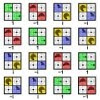# The Developer’s Cry

Yet another blog by a hobbyist programmer

# Quaternion versus Matrix performanceWhen doing 3D graphics programming, you will be dealing with vertices, vectors, translations and rotations. OpenGL will happily do the translations and rotations for you, but in some cases you will want to do the math by yourself anyway. For example, I like keeping the orientation of an object around so I can rotate it whenever I like (like in an animation or game loop or heartbeat routine). At that point, OpenGL is not involved. Later, a drawing routine is invoked that calls OpenGL to do the necessary rendering to display.

OpenGL uses matrices to represent 3D space in memory, so it makes sense to store the orientation of the object in a matrix as well. The 3D space is actually represented in a 4x4 matrix, which is generally written as an array of 16 floats. Now, there are some gotchas like the column major format to layout the matrix in memory and the right-handedness rule, but once you got that right, all the drawing routine really has to do call `glMultMatrix()` on your ‘matrix’ array of float values, and the object will (hopefully, if you got everything else right as well) appear under the desired angles. That’s easy.
Multiplying matrices together to make combined rotations is not an easy task and takes 4x16 = 64 floating point multiply operations! There are 4x12 = 48 add/subtract operations as well, but I assume that the multiplications have the worst impact on performance. If you want to do a rotate in a `glRotate`-style you will have to setup the matrix first, which adds another 24 multiply operations in the worst case, without counting the multiplications and the `sqrt()` call needed to normalize the vector.

There is another way of storing orientation, and it’s the quaternion. This is something like a vector in complex 4D space and consists of “something like” a `<x,y,z>` vector and an additional `w` component. What, only 4 values? Yup, that’s all. The memory footprint of a quaternion is really small compared to that of a matrix. (By the way, this is a non-issue to me because modern computers have plenty of memory—even the mobile devices do. But maybe you have some whack project in which you want keep tons of different orientations and memory becomes a problem). Because there are only 4 values, initializing a quaternion is dirt cheap in terms of CPU usage. Multiplying quaternions is also relatively cheap with only 16 floating point multiply operations. There are 12 add/subtract operations as well, but I assume that the multiplications have the worst impact on performance. Again, not counting the operations needed to normalize the rotation vector.
So, are quaternions the golden egg? Well, yes and no. Yes, they are great, but the main drawback is that OpenGL works with matrices. Converting the quaternion back to a matrix costs 27 multiplies.

In my book (I keep a little black book to pen down these kinds of numbers) 16+27 = 43 is still less than the 88 that matrices cost. However, there is a special case where matrices will still be faster. The trick is that when working with matrices, initially, you will have the identity matrix. Since multiplying the identity matrix with another matrix equals that other matrix (check this yourself, it’s fun …), you can greatly optimize the first matrix rotation, as it requires no multiply at all. This requires that you keep a flag on the matrix saying that it is identity. Or you can make a separate routine that simply initializes the matrix in its first rotated state. It makes for an embarrassingly fast rotate call, especially if you were going to do just one rotation of the object.
Of course, this is cheating. You can cheat in a similar way with quaternions, saving an extracted copy of its corresponding matrix and flagging it as dirty whenever it needs to be updated. Just keep in mind that if you rotate the object all the time, you will have to extract the matrix for use with OpenGL all the time.

I want to end this post with a couple of remarks:

1. Quaternions are apparently terrific for combined rotations. If you hardly do combined rotations, matrices will be faster. If you do combined rotations all the time (like for animating skeletons and such) then you probably already knew that quaternions are the way to go.
2. Quaternions produce less floating point drift than matrices, because they do less multiply operations than when multiplying matrices. They do drift however, and don’t let anybody tell you that they don’t.
3. In my post I made a remark about modern computers having enough memory (“640K ought be enough for anybody …”). The same goes for CPU power, really. However, on mobile devices it probably does pay off to investigate app performance not only because of the less powerful CPU, but also because of battery power consumption.
4. I got to writing this blog entry because I spent a day wondering why my matrix rotation around an arbitrary axis gave weird results. The quaternion code did work, until I passed in a vector that was not normalized and it displayed the exact same weird result! That was an eye-opener. After normalizing said vector, the matrix code gave just as good results.
5. Over a year ago, when I wrote in my blog about quaternions for the first time, I made a remark that NeHe’s code has a sign wrong somehow. To my surprise, my model was rotating clockwise using my own quaternion code. I fixed it to have it rotate anti-clockwise. Either I got the matrix column major layout wrong before, or my other project was working with different axis. Anyway, NeHe’s quaternion code is probably alright after all. I didn’t use it. By the way, I saw wikipedia too now shows code examples for quaternions.